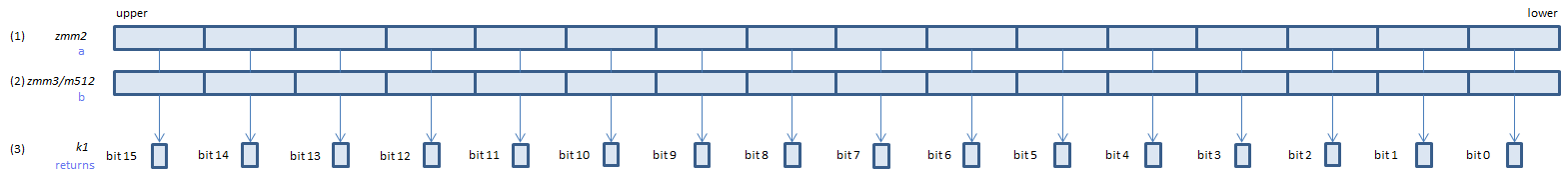﻿ cmpeqps

## CMPEQPS - CoMPare EQual Packed Single

CMPEQPS xmm1, xmm2/m128    (S1
__m128 _mm_cmpeq_ps(__m128 a, __m128 b)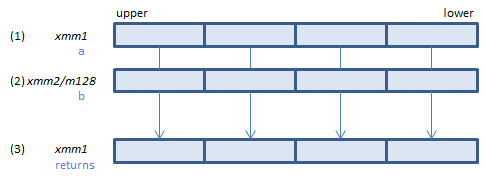For each float, if (1) == (2) set 1, else set 0, to all bits of the corresponding float of (3).
VCMPEQPS xmm1, xmm2, xmm3/m128    (V1
__m128 _mm_cmpeq_ps(__m128 a, __m128 b)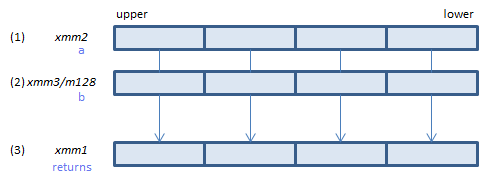For each float, if (1) == (2) set 1, else set 0, to all bits of the corresponding float of (3).
VCMPEQPS ymm1, ymm2, ymm3/m256    (V1
__m256 _mm256_cmp_ps(__m256 a, __m256 b, _CMP_EQ_OQ)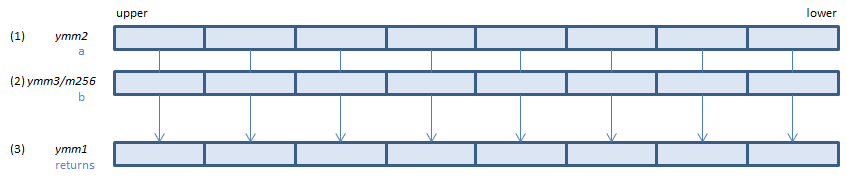For each float, if (1) == (2) set 1, else set 0, to all bits of the corresponding float of (3).
VCMPEQPS k1{k2}, xmm2, xmm3/m128/m32bcst    (V5+VL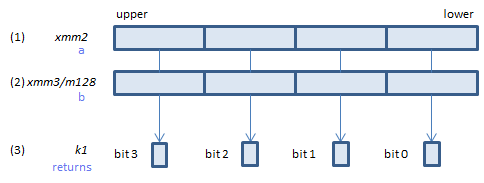For each float, if (1) == (2) set 1, else set 0, to the corresponding bit of (3).
If k2 bit is 0, the comparison is not done and the corresponding bit of (3) is set to zero. Upper bits of (3) are zero cleared.
VCMPEQPS k1{k2}, ymm2, ymm3/m256/m32bcst    (V5+VL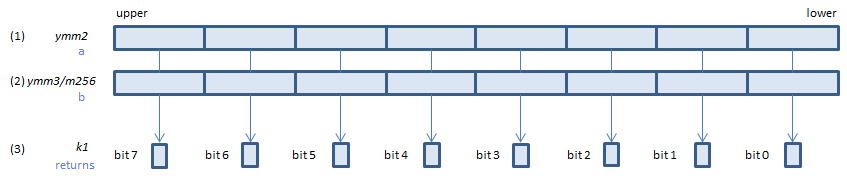For each float, if (1) == (2) set 1, else set 0, to the corresponding bit of (3).
If k2 bit is 0, the comparison is not done and the corresponding bit of (3) is set to zero. Upper bits of (3) are zero cleared.
VCMPEQPS k1{k2}, zmm2, zmm3/m512/m32bcst{sae}    (V5